# Fractional clique decompositions of dense graphs and hypergraphs

Together with Daniela Kühn, Allan Lo and Deryk Osthus I proved that for every graphthere is a constant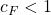such that every “-divisible” graph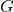on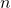vertices with minimum degree at least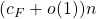has an-decomposition. In practice, the current obstacle to improving the bounds on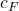is usually our knowledge of another quantity, the fractional decomposition threshold for cliques.

A graphhas a fractional-decomposition if we can assign a non-negative weight to each copy ofinsuch that the total weight of the copies ofcontaining each fixed edge ofis exactly 1. We prove that every graph with minimum degree at least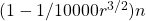has a fractional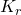-decomposition. This greatly improves the previous bound of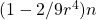for large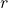. We also prove a similar result for hypergraphs.

The proof begins with an approximate fractional-decomposition obtained by weighting every-clique in our graph equally. We then use small gadgets to make local adjustments to the total weight over each edge until we end up with a genuine fractional-decomposition.